Latest Banking jobs   »

# Reasoning Ability Quiz For IDBI AM/Executive 2022- 13th June

Directions (1-3): Study the following information carefully and answer the questions given below.
A&B (5) – A is 10m North of B
A%B (20) – A is 40m South of B
A@B (10) – A is 20m East of B
A#B (15) – A is 30m West of B

P&Q (12), R%P (5), S#R (6), T%S (9), U@T (8), V@P (4)

Reasoning Quiz For IDBI AM/Executive 2022 13th June CHECK IN HINDI

Q1. What is the direction of U with respect to P?
(a) South-East
(b) North-East
(c) North-West
(d) None of these
(e) South-West

Q2. What is the shortest distance between R and V?
(a) 50m
(b) 25m
(c) 36m
(d) 2√41m
(e) 3√56m

Q3. If X is the midpoint of the line formed between S and T, then what will be the distance between X and S?
(a) 15m
(b) 18m
(c) 9m
(d) 10m
(e) None of these

Directions (4-6): Study the following information carefully and answer the questions given below.
Point Y is 20m north of point Q. Point S is 7m east of point Q. Point Z is 17m north of point U. Point U is 7m west of point Q. Point X is 9m south of point Z. Point S is 9m north of point R. Point Y is 5m east of point T. Point A is towards the south of point U and west of point R respectively.

Q4. What is the shortest distance between point X and point R?
(a) √485m
(b) √486m
(c) 22m
(d) √487m
(e) None of these

Q5. Point Z is in which direction with respect to point S?
(a) North-East
(b) North-west
(c) East
(d) South
(e) None of these

Q6. What is the direction of Point T with respect to point X?
(a) South-east
(b) South-west
(c) North-east
(d) North-west
(e) None of these

Directions (7-9): Read the following information carefully and answer the questions which follow.
Point B is 10m to the north of point W which is 7m to the west of point E. Point D is 5m to the south of Point E. Point P is 9m to the east of Point B. Point E is the midpoint between Point W and Point C, point C being towards the East of point W. Point H is 4m to the north of Point A. Point W lies exactly between Point E and Point A, point A being towards the West of point W.

Q7. What is the direction of Point B with respect to Point D?
(a) North-east
(b) North-west
(c) South-east
(d) South-west
(e) None of these

Q8. If point N is 5m south of point W, then what is the total distance between Point N and Point C, also Point N is in which direction from Point H?
(a) 22m, north-west
(b) 24m, north-east
(c) 19m, south-east
(d) 15m, north
(e) None of these

Q9. Which of the following points lie in the straight line?
(a) B, W and E
(b) H, A and P
(c) W, E and D
(d) A, W and C
(e) None of these

Directions (10-12): Study the information carefully and answer the questions accordingly.

Mahesh walked 10m south from point C and reached point D. Now, he took a left turn and walked 14m to reach point E. Now, he took another left turn and walked 12m to reach point F. Then he took a left turn and walked 6m to reach point G. Finally, he took another left turn and walked 16m and reached point H.

Q10. Point H is in which direction of point E?
(a) South-West
(b) North-East
(c) West
(d) South
(e) None of these

Q11. Point G is in which direction of point D?
(a) South-West
(b) North-East
(c) West
(d) South
(e) None of these

Q12. Four among the following five are same in a certain way and forms a group. Which among the following does not belong to the group?
(a) C, G
(b) D, F
(c) H, E
(d) G, H
(e) C, F

Directions (13-15): Study the following information carefully and answer the questions given below.
Shalini is going to the market from her home. She moves 8m in the north and then turns left and moves 5m, now she turns right and moves 9m to reach her friend’s house, then again she turns in the south direction and moves 5m and then she turns left and moves 5m to reach the market.

Q13. What is the shortest distance between her home and market?
(a) 10m
(b) 9m
(c) 12m
(d) 8m
(e) None of these

Q14. If her uncle’s house is in line with her home but north east of her friend’s house, then what can be the distance between her home and her uncle’s house?
(a) 19m
(b) 8m
(c) 11m
(d) 17m
(e) 15m

Q15. In which direction is her friend’s house with respect to her home?
(a) North – west
(b) South
(c) South – east
(d) West
(e) Can’t be determined

Solutions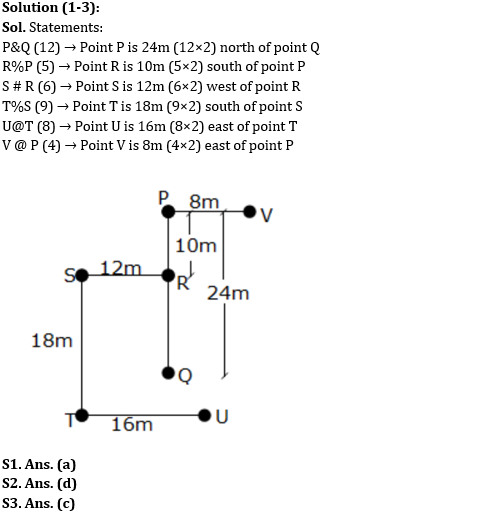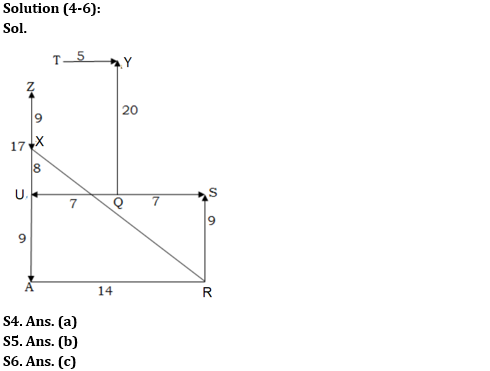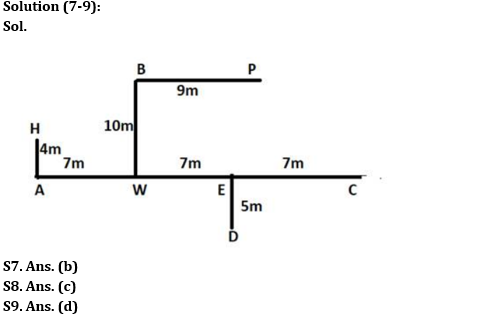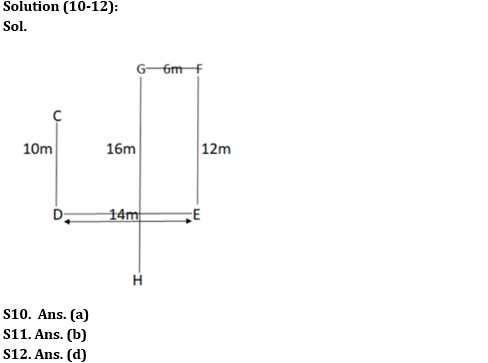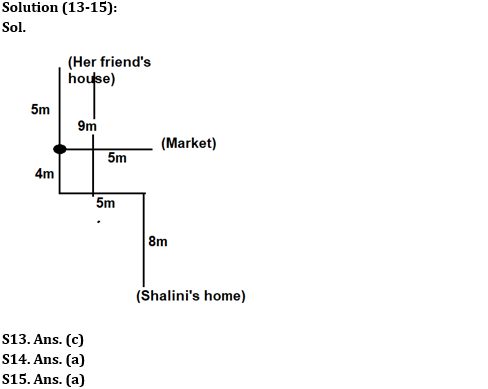#### Congratulations!